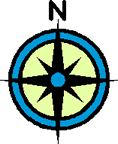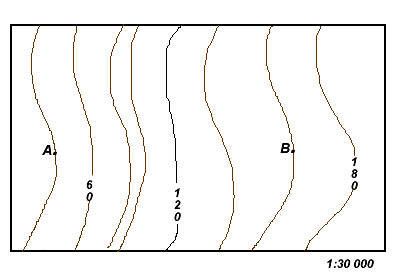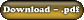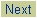Let's look at how to calculate gradient which is a measurement of the steepness of the land or simply slope.
 ExampleCalculate the gradient from A to B in m/ km, %, and ratio. Calculating The Rise Find the elevation at B and subtract the elevation at A. This will give you the change in elevation if you were to walk from Point A to Point B. Hence, B - A = 160 m - 40 m = 120 m. This is the Rise. Calculating The Run When you put your ruler down between Point A and B, there is a map distance of 6.7 cm. The scale is 1:30 000; therefore each centimetre on the map represents 0.3 km in reality. Hence, the actual distance between Point A and B is 6.7 cm x 0.3 km = 2.01 km. This is the Run. 1) Metres / km Rise = 120 m Run = 2.01 km = 60 m/km (Note: Typically in this course, you will round the m / km to the nearest whole number.) What this number simply means is that if you were to walk from Point A to Point B, for every kilometre that you traveled horizontally you would also be gaining 60 metres in elevation. 2) Percentage Rise = 120 m Run = 2.01 km Convert the bottom into kilometres. [Recall : 1 km = 1000 metres] Therefore, 2.01 km = 2010 metres. Rise = 120 m Run = 2010 m Divide the numerator (top) by the denominator (bottom) and multiply by 100 to get a percentage. Rise = 120 m Run = 2010 m x 100 = 6% (Note: Again, typically in this course, you will round the % to the nearest whole number.) Percentage is often used to express gradient on road signs which is critical information for truck drivers. 3) Ratio Rise = 120 m Run = 2010 m Reduce the fraction by dividing the top and bottom by the top number. Rise = 120 m / 120 = 1 Run = 2010 m /120= 16.8 Express this reduced fraction as a ratio for your final answer. Therefore, 1:16.8 (Note: Typically in this course, you will round the ratio to the nearest first decimal.)
 Assignment Work 1) Download, print out, and complete the Gradient Exercises.2) Check over your answers using the key.©www.coolschool.ca Press thebutton on the Action menu to proceed.